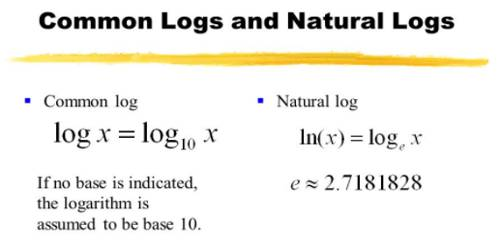In mathematics, logarithms were developed for making complicated calculations simple. In mathematics, the logarithm is the inverse operation to exponentiation, just as division is the inverse of multiplication and vice versa. That means the logarithm of a number is the exponent to which another fixed number, the base, must be raised to produce that number.

In mathematics logarithm rules or log rules, we have discussed mainly on logarithm laws along with their proof. If students understand the basic proof of general laws of logarithm then it will be easier to solve any types of questions on logarithm like-

There is four following math logarithm formulas:

• Product Rule Law: loga (MN) = loga M + loga N
• Quotient Rule Law: loga (M/N) = loga M – loga N
• Power Rule Law: IogaMn = n Ioga M
• Change of base Rule Law: loga M = logb M × loga b

Common Logarithm and Natural Logarithm

In Logarithm we have already seen and discussed that the logarithmic value of a positive number depends not only on the number but also on the base; a given positive number will have different logarithmic values for different bases.

In practice, however, following two types of logarithms are used:

(i) Natural or Napierian logarithm

(ii) Common logarithm

The logarithm of a number to the base e is known as Napierian or Natural logarithm after the name of John Napier; here the number e is an incommensurable number and is equal to the infinite series:

1 + ¹/₁₀ + ¹/₂₀ + ¹/₃₀ + ………… ∞

The logarithm of a number to the base 10 is known as the common logarithm.

This system was first introduced by Henry Briggs. This type is used for numerical calculations. The base 10 in common logarithm is usually omitted.

For example, log₁₀ 2 is written as log 2.

The rest of the part deals with the method of determining common logarithms of positive numbers.Characteristic and Mantissa:

Now, consider a number (say 6.72) between 1 and 10. Clearly,

1 < 6.72 < 10

Therefore, log 1 < log 6.72 < log 10

or, 0 < log 6.72 < 1 [ Since log 1 = 0 and log 10 = 1]

Therefore, the logarithm of a number between 1 and 10 lies between 0 and 1. That is,

log 6.72 = 0 + a positive decimal part = 0∙ …………..

We now consider a number (say 58.34) between 10 and 100. Clearly,

10 < 58.34 < 100

Therefore, log 10 < log 58.34 < log 100

or, 1 < log 58.34 < 2 [Since log 10 = 1 and log 100 = 2 ]

Therefore, the logarithm of a number between 10 and 100 lies between 1 and 2. That is,

log 58.34 = 1 + a positive decimal part = 1∙ ……

Similarly, the logarithm of a number (say 463) between 100 and 1000 lies between 2 and 3 (since log 100 = 2 and log 1000 = 3). That is,

log 463 = 2 + a positive decimal part = 2∙ …….

In like manner the logarithm of a number between 1000 and 10000 lies between 3 and 4 and so on.

Now, consider a number (say .54) between 1 and .1. Clearly,

.1 < .54 < 1

Therefore, log .1 < log .54 < log 1

or, – 1 < log .54 < 0, [Since log 1 = 0 and log .1 = – 1]

Therefore, the logarithm of a number between .1 and 1 lies between – 1 and 0. That is,

log .54 = -0∙ ……. = – 1 + a positive decimal part.

We now consider a number (say .0252 ) between .1 and ∙01. Clearly,

.01 < .0252 < .1

log 0.1 < log .0252 < log .1

or, -2 < log .0252 < – 1 [since log .1 = – 1 and log .01 = -2]

Therefore, the logarithm of a number between .01 and .1 lies between -2 and – 1 . That is,

log .0252 = – 1∙ ….. = – 2+ a positive decimal part.

Similarly, the logarithm of a number between .001 and .01 lies between – 3 and -2 and so on.

From the above discussions, it is observed that the common logarithm of a positive number consists of two parts. One part is integral which may be zero or any integer (positive or negative) and the other part is non-negative decimal.

The integral part of a common logarithm is called the characteristic and the non-negative decimal part is called the mantissa.

Suppose, log 39.2 = 1.5933, then 1 is the characteristic and 5933 is the mantissa of the logarithm.

If log .009423 = – 3 + .9742, then – 3 is the characteristic and .9742 is the mantissa of the logarithm.

Since log 3 = 0.4771 and log 10 = 1, so the characteristic of log 3 is 0 and the mantissa of log 10 is 0.

Information Source: